# Open circuit test & Short circuit test in transformers

Open circuit test and short circuit test are conducted to determine the core loss, copper loss, and equivalent circuit parameters of a transformer. In this article, in addition to the tests mentioned in the title, we will also discuss the polarity test and Sumpner’s test.

Normally transformers are tested at no-load. Transformers can be tested by connecting load to its secondary if its rating is small. In the case of large transformers, it is exceedingly difficult to arrange large loads enough for direct loading. Also, load tests are associated with a large amount of power wastage. Hence it is preferred to test transformers without load.

## Why is it necessary to conduct OC and SC tests?

It is necessary to know the impedance of the transformer in order to calculate its voltage regulation and efficiency. The impedance and other circuit parameters can be determined by conducting simple no-load tests. No-load tests have a minimal power loss when compared to that during direct load tests.

The input voltage, current, and power are measured and based on that the equivalent circuit parameters can be determined.

## Open circuit test

The open circuit test is performed to determine the no load losses or core losses as well as the turns ratio, no load currents, magnetizing components and core loss components of the transformer.

### Circuit for open circuit test

For convenience, the supply is connected to the LV side of the transformer and the HV side of the transformer is left open. Voltmeters, ammeters and watt meter are connected as shown in the figure below.

### Open circuit test Procedure

• Apply rated voltage to the LV side of the transformer.
• Measure the no-load current Io, power Po, and input voltage Vo.
• Measure the open-circuited HV side voltage if the transformer ratio needs to be calculated.

### Calculation of core losses and magnetizing components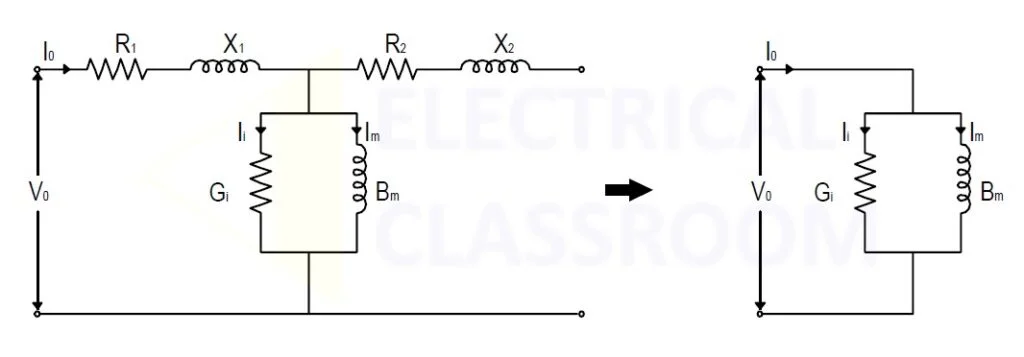As no load is connected to the secondary, the current flow and the losses due to winding resistance and reactance are very less and can be neglected and the circuit is simplified.

Neglecting the copper loss, we calculate the core losses and the core loss components.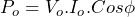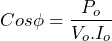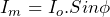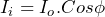Where, Im is the magnetizing current and Ii is the core loss component.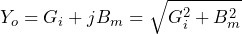Also,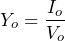The conductivity of the core,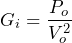The Susceptance of the core,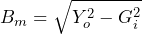Where Y0 is the core admittance, Gi is the conductivity of the core, Bm is the Susceptance of the core.

## Short Circuit test

The purpose of conducting a short circuit test is to determine the winding resistance, reactance, and the copper loss of the transformer.

### Circuit for short circuit test

For convenience a variable voltage source is connected to the HV side of the transformer and the LV side of the transformer is short circuited. This is because, the voltage required for short circuit test is typically 5 per cent of the rated value. Since the current rating of HV is less than the LV, the current drawn at 5 per cent of rated voltage of HV is low. At the same time, if we apply voltage to LV winding, the current drawn from the supply will be very high. It is difficult to arrange a low voltage – high current power source.

### Short circuit test Procedure

• Gradually raise the supply voltage from zero, until the transformer draws its rated current.

It can be noted that the applied voltage, Vsc, required to circulate current Isc is very small compared to the rated voltage of the winding (typically 5% of rated voltage). Therefore, the excitation current required is too small and can be neglected.

The power input to the transformer measured by Psc corresponds to copper loss. Therefore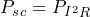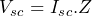Where,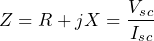The resistance offered by the coil,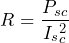The Susceptance of the core,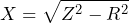The attained values of R and X are referred to the HV side of the transformer from which the test is conducted. If can be referred to the other side using the operator a2 (square of turns ratio).

## Calculation of transformer efficiency

If P0 and Psc are the core loss and the copper loss of a transformer respectively, the efficiency of the transformer can be calculated using the following formula: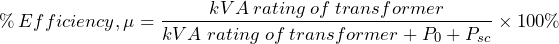## Polarity test

Polarity test is conducted to find the polarity of the transformer windings. Those ends of the two transformers that acquire positive or negative polarity simultaneously are said to be at similar polarity.  It is necessary to know the polarity of the winding in order to connect it properly.

### Method 1:

• One end of the secondary A1 is connected to an end of the primary winding (say a1).
• Connect a voltmeter across the other two ends (a2 and A2).
• Now apply a voltage across the primary winding a1 and A1.

Now, if the voltage read by the voltmeter is V1~V2 then the polarities of A1 and a1  is the same and if the voltage read is V1 + V2 then polarities of A1 and a2  is the same.

### Method 2:  DC flashing method

DC flashing test is used while the transformer is tested on the field. This method involves the usage of a DC battery of 1.5 to 6V and a DC voltmeter (Permanent magnet type). The circuit to be made is shown in the below figure.  The battery and the switch are connected to the HV side of the transformer.

When the switch is closed, the end of the LV winding that is at the same polarity as that of the HV winding to which the positive terminal of the battery connected becomes positive (This is indicated by the deflection of the DC voltmeter). When the switch is opened, the similar polarity end acquired negative potential.

## Sumpner’s test

Sumpner’s test, otherwise known as “back-to-back test”, is a method to determine the steady-state temperature rise of the transformer. This test is conducted by connecting two similarly rated transformers back to back.

### Circuit for Sumpner’s test

The primaries of two transformers are connected in parallel while their secondaries are connected in series, but in phase opposition such that the voltage across the terminal is zero. To connect them in phase opposition, polarities of the winding should be known. Hence, the polarity test can be conducted if required.

Since the secondaries are connected in phase opposition, no current shall flow through it even if they are short-circuited and the current measured by the ammeter A1 corresponds to the core loss component.

Similarly when the primaries are short-circuited, full current flows through the circuit. The current measured by W2 corresponds to the copper loss of the transformer.

Thus by conducting Sumpner’s test, we can calculate the iron and copper losses without even loading the transformer. The total power input to the transformer is (2Po + 2Pc).

The steady-state temperature rise of the transformer can be monitored by conducting this test for a longer period and monitoring the temperature rise using a thermometer.